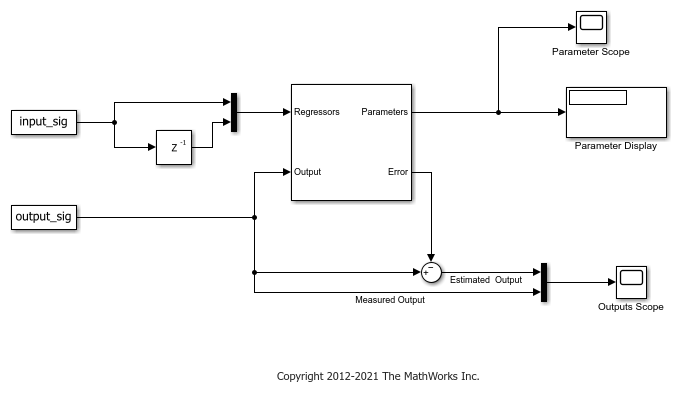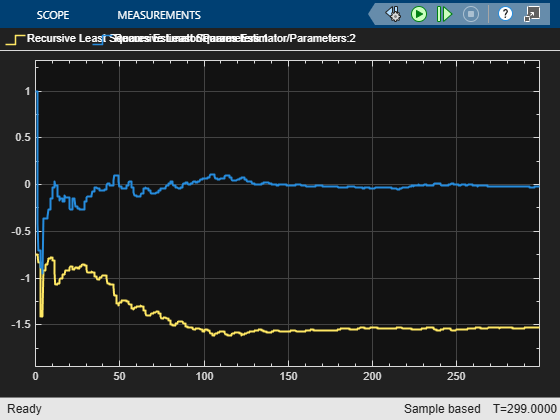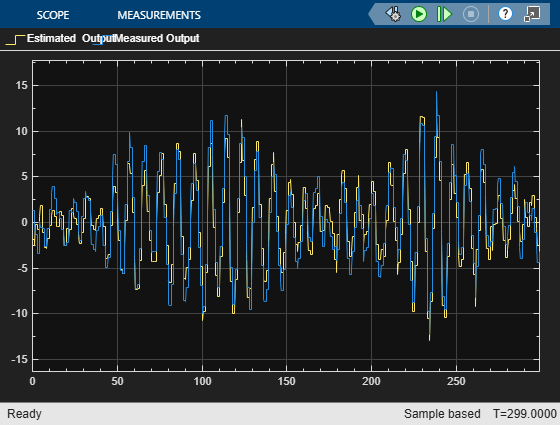Main Content

# Estimate Parameters of System Using Simulink Recursive Estimator Block

This example shows how to estimate the parameters of a two-parameter system and compare the measured and estimated outputs.

This example is the Simulink version of the command-line parameter-estimation example provided in recursiveLS.

The system has two parameters and is represented as:Here,

•andare the real-time input and output data, respectively.

•andare the regressors,, of the system.

•andare the parameters,, of the system.

Load the data and extract the input, output, and time information.

To directly compare this example with the command-line recursiveLS example, shift the time vector by one position. This shift synchronizes the Simulink input (time starts at 0) with the command-line input (time starts at the first sample time).

load iddata3 input = z3.u; output = z3.y; time = z3.SamplingInstants-1; 

Construct Input and Output signals for Simulink.

input_sig = timeseries(input,time); output_sig = timeseries(output,time); 

Open a preconfigured Simulink model based on the Recursive Least Squares Estimator block. In this model:

• The input_sig and output_sig blocks import input_sig and output_sig.

• The model constructs the Regressors signal by routing input_sig through a delay-line block, and then multiplexing the delayed signal with the original signal.

• An Error port provides the estimated error signal. A Sum block subtracts this error from input_sig to produce the estimated output.

• A Mux block then combines the measured (output_sig) and estimated output signals so that you can view them together on the scope.

rls = 'ex_RLS_Estimator_Block_sb_inf'; open_system(rls)Run the simulation. The Parameter Scope shows the progression of the estimation. The adjacent Parameter Display displays the final numerical values. The Outputs Scope plots the measured and estimated outputs together.

sim(rls) open_system([rls '/Parameter Scope']) open_system([rls '/Outputs Scope'])You can explore other block configurations by modifying the model parameters. For instance,

• Change Estimation Method to see how your algorithm choices affect your results.

• Change History to finite and your Window Length to 30 to apply the sliding-window algorithm.

## SupportGet trial now